The Minoids Applet.
Run it!
Instructions
Java Sources
Screenshots
Sample Report

The Polyominoids

The polyominoids are polyforms composed by connected minoids. A minoid is any cube's face inside a cubic lattice. The polyominoids are a yet another polyominoes super set. These are valid polyominoids: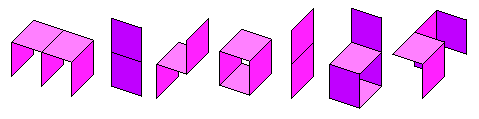The Minoids inside the cubic lattice

A cubic lattice contains vertices, edges, faces (minoids) and cubes as geometric elements. In the three dimensional space, triplets of whole numbers are enough to identify each geometric element from the rest. According the coordinates values' parity we can classify the elements in this way:
ElementCoordinates
ODD
Coordinates
EVEN
Dot Color in
the lattice
Points03Blue
Lines12Magenta
Minoids21Red
Cubes30Black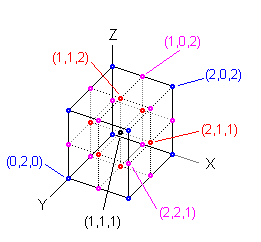The colored dots represents the geometric center of the elements. For instance, the magenta dots are the midpoints of the Lines of the lattice, while the red dots are the centers of the minoids. Is important to note that the numbers of the table holds for the three-dimensional space. A minoid inside the four-dimensional space needs 4 coordinates 2 odd and 2 even and inside the five-dimensional space needs 5 coordinates 2 odd and 3 even..., that is, always needs 2 odd coordinates and the rest even. Look this isocolored minoids having same x at the left, same y at the center, and same z at the right: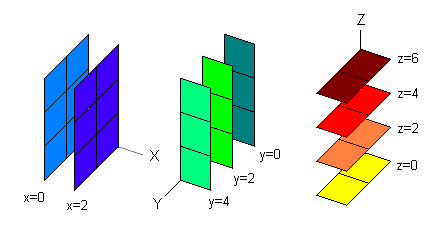BoxesA box Box(a,b,c) is the set of all minoids with coordinates satisfying these conditions:

0 <= x <= 2a
0 <= y <= 2b
0 <= z <= 2c

The figure at the right shows the Box(1,2,3): The box shown is separated into three sections. The cyan section has minoids with coordinate x=0, the green section has minoids with coordinate x=1 and the yellow section has minoids with coordinate x=2.

The numbering convention is such that permits to have an incrementing pattern based in signification, being the x coordinate, the first of all dimensions, the Most Significant Coordinate. In the figure first minoid, the number 1, has coordinates (0, 1, 1), minoid 2 has coordinates (0, 1, 3) and so on. The last minoid, the 29, has coordinates (2, 3, 5).

Minoids bounded by a Box

The minimum order of a Box is defined as the minimum n-minoid which needs the box to be bounded. An empirical formula follows, though obvious. For the Box(1,2,3) the minimum order is:

a + b + c - 1 = 5

The maximum order is simply the total number of minoids of the box. For the Box(1,2,3) the maximum order is:

ab(c+1) + a(b+1)c + (a+1)bc = 29

This minimum and maximum orders of the Box(1,2,3) indicates this box is the bounding box for some of the 5, 6, 7, ... , 28, 29-minoids. Both, the cyan and yellow sections (x=0, x=2) of the Box must have at least 0 minoids and at most b*c = 6 minoids. The green section (x=1) of the Box(1,2,3) must have at least 1 minoid and at most b(c+1) + (b+1)c = 17 minoids.

 These are all the translations' boxes for bounding all the dominoids. This java code creates all the translations boxes given the minoid order.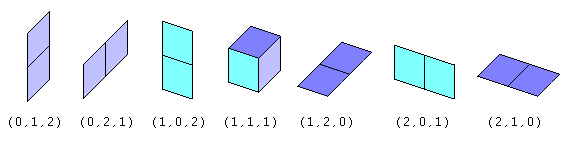These are all the rotations' boxes for bounding all the tetrominoids. This java code creates all the rotations boxes given the minoid order.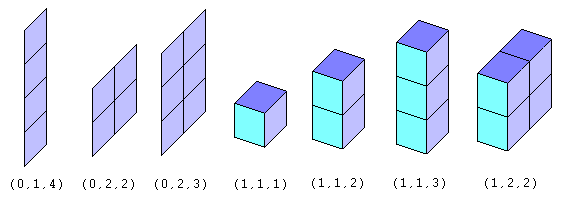Minoids Connections

The polyominoes, as you should know, connects their monominoes in only one way: a square attached next to another square, being the squares in the same plane. In minoids terminology we call this connection is soft or weak. The polyominoids connects the monominoids in two distinct ways, one the mentioned soft and another new we call hard or strong: This java code reports valid connections between minoids.

 The four Soft Connections The eight Hard Connections The twelve All Connections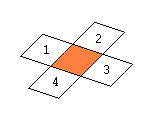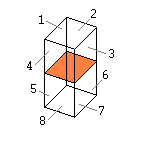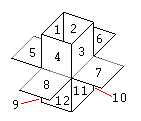The soft and hard connections are shown in the next figures. The coordinates of the minoids and the coordinates of the line connections are included.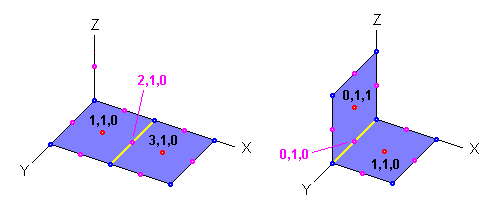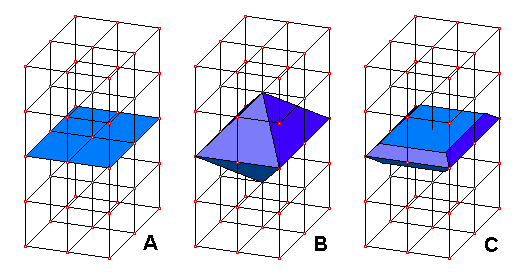The soft connections are 'weak' bounds between the minoids, since is difficult to get physical minoids mantain rigidity with soft connections. In the other hand, the hard connections are 'strong' bounds between the minoids because is possible to get physical thick minoids with hard connections.

Three steps at the right describe how to construct a real minoid.

• Figure A shows the ideal minoid inside the unit lattice.
• Figure B shows how the minoid is expanded into an octahedron. This octahedron fills completly the space, and is capable to connect to other octahedra by its faces. This is exactly the hard connection. The octahedron face is an isocelles triangle with 2 units base and the other two sides of length square root of 3.
• Figure C shows the octaedra truncated from the north and south vertices in such a way we get a thick minoid capable to holding hard connections. The final body is a decahedron. The connection is made attaching two minoids by their trapezoidal faces, leaving the square face always free. Then, the eight trapezoids represents the eight possible hard connectors of the minoid.

The two types of connections (soft and hard) give three types of polyominoids:

Soft polyominoids
Technically these polyominoids are just polyominoes, e.g. ALL minoids connections in polyominoes are 'soft', the only permited connection. Examples: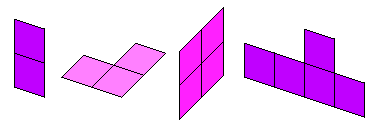Hard polyominoids
Are those polyominoids (no polyominoes) having ALL minoids connections 'hard'. This polyominoids can be constructed physically with the decahedron mentioned above. All the constructions are totally rigid. Examples: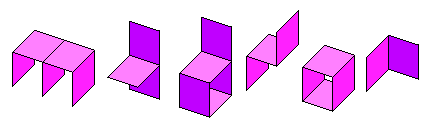Mixed polyominoids
Are those polyominoids (no polyominoes) having at least one connection 'soft' and at least one connection 'hard'. Since the soft connection required, the constructions are not totally rigid, except in few cases we are going to mention.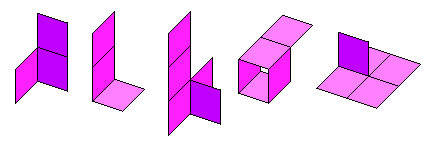Look the next pentominoid, is rigid. Even though minoids 4 and 5 are soft connected, at the same time, they are hard connected too, through the rest of the minoids 1, 2 and 3. Is hard or mixed connected? The actual software routines say is mixed, then the rigidity must be figured out by other means.: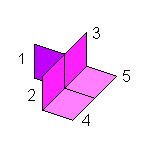Translations Polyominoids Counting

The applet software routines counts the minoids differently:
1. Counts All the polyominoids, that is Total = Mixed + Hard + Soft
2. Counts only the hard ones separately.
3. Counts the soft ones separately.
In order to get the mixed polyominoids, is necessary to extract the pures hard and soft polyominoids from the all set:

Mixed = All - Hard - Soft

The counting is in this way since there are computed bits mask to detect minoids connections, pair by pair. The masks used are of three types: any, hard or soft, and are figured out before the counting takes place.

Every 5-minoid inside the Box(1,2,3) is represented as a set of 29 bits, 5 ones and 24 zeros. First an incrementing process takes place to move the 5 bits inside the 29 bits space. This reach the total number of testings to the binomial coefficient of 29 over 5, or

29*28*27*26*25 / 1*2*3*4*5 = 118,755

...instead of working wiht the very big number of 229 of ordinary binary counting.

The 118,755 resulting 5-ones numbers are compared against 6 previouly prepared masks to determine if the suspected pentominoid reaches all the walls of the box for fullfill with the boundings. This java code performs the binomial coefficients incremental machine and checks if the polyominoid bits number satisfy the 6 bounding conditions.

Finally the polyominoids fullfilling the bounding are tested for their total connectivity of the 5 minoids, whether any, hard or soft connection were selected in turn. This java code checks if the already bounded polyominoid bits number satisfy a particular connectivity type.

Minoids' interesting Java Code

Next code parts taken from the source java classes are the main algorithms for the minoids. Some of the above sections refer to them. The whole code is in classes and packages. Happy hacking!.

This java code reports valid connections between minoids:
 ``` public boolean isAnyConnected(Minoid other) { return (isHardConnected(other) || isSoftConnected(other)); } public boolean isHardConnected(Minoid other) { dx = Math.abs(x - other.x); dy = Math.abs(y - other.y); dz = Math.abs(z - other.z); if (dx == 0 && dy == 1 && dz == 1) return true; if (dx == 1 && dy == 0 && dz == 1) return true; if (dx == 1 && dy == 1 && dz == 0) return true; return false; } public boolean isSoftConnected(Minoid other) { dx = Math.abs(x - other.x); dy = Math.abs(y - other.y); dz = Math.abs(z - other.z); if ((x & 1) == 0 && dx == 0) { if ((dy == 2 && dz == 0) || (dy == 0 && dz == 2)) return true; } else if ((y & 1) == 0 && dy == 0) { if ((dx == 2 && dz == 0) || (dx == 0 && dz == 2)) return true; } else if ((z & 1) == 0 && dz == 0) { if ((dx == 2 && dy == 0) || (dx == 0 && dy == 2)) return true; } return false; } ```

Jorge Luis Mireles Jasso
October 20, 2001
Torreón, Coah. México

Also in this site:

Hosted by www.Geocities.ws